Next: Robot synthesis example Up: Examples of applications of Previous: Robot singularity analysis   Contents

Robot workspace analysis

For the robot presented in the previous examples we have a set of parameters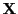that allow to represent the position/orientation of the platform. At the same time we have various geometrical parameters that describe the possible geometry of the robot. Examples of the these parameters are the distance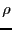between the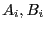, the angle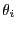between the vector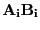and a fixed direction in space (this angle represent the amplitude of the motion of the joint we have at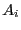).

In practice all the geometrical parameters are submitted to constraints. For example the distancesmust satisfy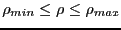, the anglemust be such that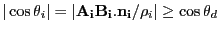where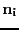is a unit vector that describes the main orientation of the ball-and-socket joint atand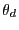is the maximal possible rotation of this joint. All these constraints imply that the value of the elements ofare restricted to lie in a specific region embedded in the 6-dimensional space, this region being called the reachable workspace of the robot as the points of this region define all the position/orientation that can be reached by the platform.

In some cases it is possible to find an analytical description of the workspace (for example if the orientation is fixed and only the distance constraints are taken into account) but as soon as all the constraints are taken into account such analytical form is difficult to obtain. Interval analysis allows to obtain an approximation of the workspace as a set of 6-dimensional boxes. It is only an approximation as the algorithm, based on a classical bisection method, discard boxes whose width is lower than a given threshold. At the same time it is possible to obtain a bound on the error between the volume of the approximation and the volume of the workspace. For more details see . A variant of the algorithm allows to solve efficiently a very practical problem: is a given region included in the reachable workspace of the robot ?Next: Robot synthesis example Up: Examples of applications of Previous: Robot singularity analysis   Contents
Jean-Pierre Merlet 2012-12-20